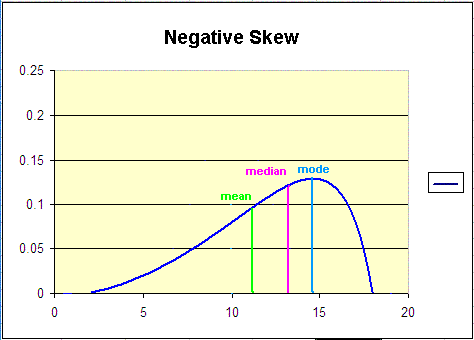# Mean, Median, Mode, and Range

Before you can begin to understand statistics, there are four terms you will need to fully understand. The first term 'average' is something we have been familiar with from a very early age when we start analyzing our marks on report cards. We add together all of our test results and then divide it by the sum of the total number of marks there are. We often call it the average. However, statistically it's the Mean!

The Mean

Example:

Four tests results: 15, 18, 22, 20
The sum is: 75
Divide 75 by 4: 18.75

The 'Mean' (Average) is 18.75

(Often rounded to 19)

The Median
The Median is the 'middle value' in your list. When the totals of the list are odd, the median is the middle entry in the list after sorting the list into increasing order. When the totals of the list are even, the median is equal to the sum of the two middle (after sorting the list into increasing order) numbers divided by two. Thus, remember to line up your values, the middle number is the median! Be sure to remember the odd and even rule.

Examples:

Find the Median of: 9, 3, 44, 17, 15 (Odd amount of numbers)
Line up your numbers: 3, 9, 15, 17, 44 (smallest to largest)
The Median is: 15 (The number in the middle)

Find the Median of: 8, 3, 44, 17, 12, 6 (Even amount of numbers)
Line up your numbers: 3, 6, 8, 12, 17, 44
Add the 2 middles numbers and divide by 2: 8 12 = 20 ÷ 2 = 10
The Median is 10.

The Mode

The mode in a list of numbers refers to the list of numbers that occur most frequently. A trick to remember this one is to remember that mode starts with the same
first two letters that most does. Most frequently - Mode. You'll never forget that one!

Examples:

Find the mode of:
9, 3, 3, 44, 17 , 17, 44, 15, 15, 15, 27, 40, 8,
Put the numbers is order for ease:

3, 3, 8, 9, 15, 15, 15, 17, 17, 27, 40, 44, 44,
The Mode is 15 (15 occurs the most at 3 times)

*It is important to note that there can be more than one mode and if no number occurs more than once in the set, then there is no mode for that set of numbers.

Ocasionally in Statistics you'll be asked for the 'range' in a set of numbers. The range is simply the the smallest number subtracted from the largest number in your set. Thus, if your set is 9, 3, 44, 15, 6 - The range would be 44-3=41. Your range is 41.This is a question game to help you learn Mean, Median, Mode and Range.
Here is a site with a practice sheet on it to see if you are doing each term correctly.
There are a lot of frequently asked questions about the Mean, Median, Mode and Range. Go to this site to have some of them answered.